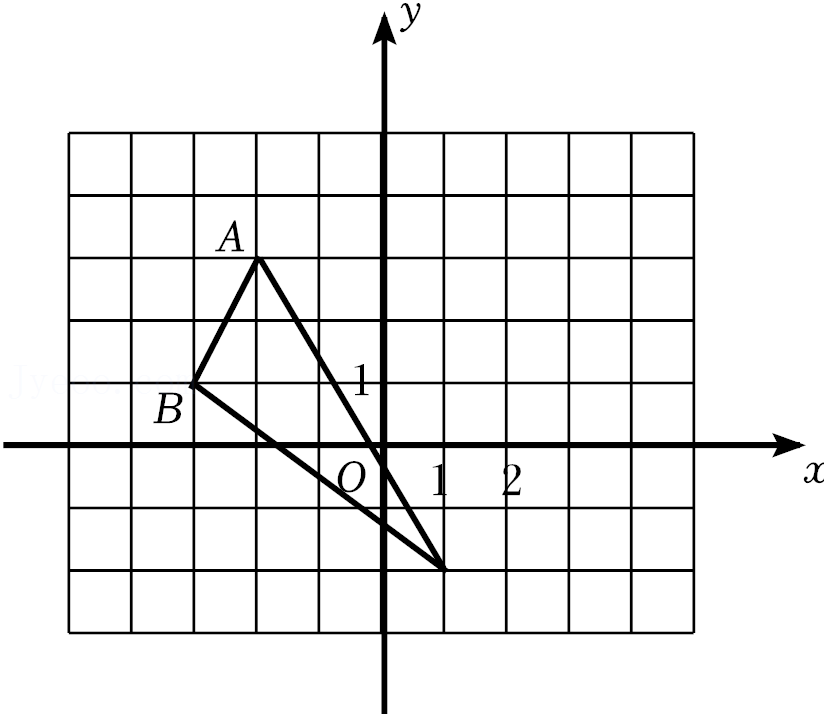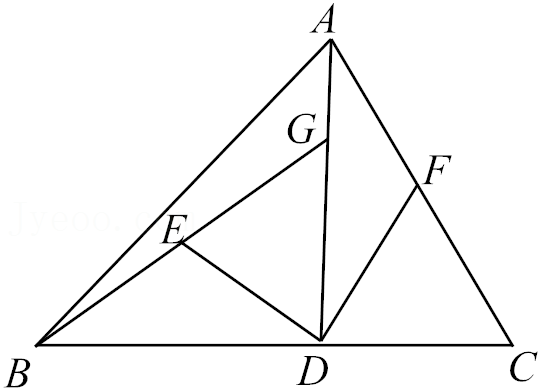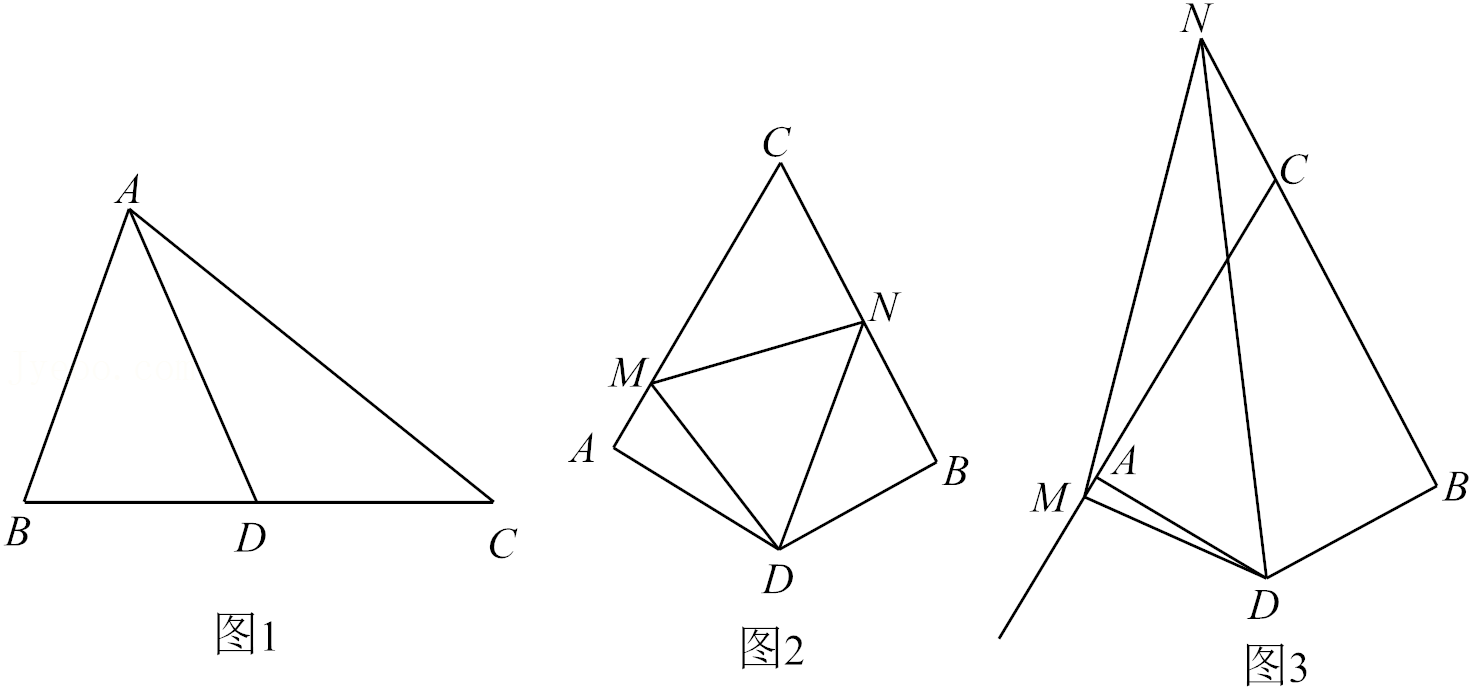【2021-2022学年山东省潍坊市八年级（上）期中数学试卷】-第1页 试卷格式：2021-2022学年山东省潍坊市八年级（上）期中数学试卷.PDF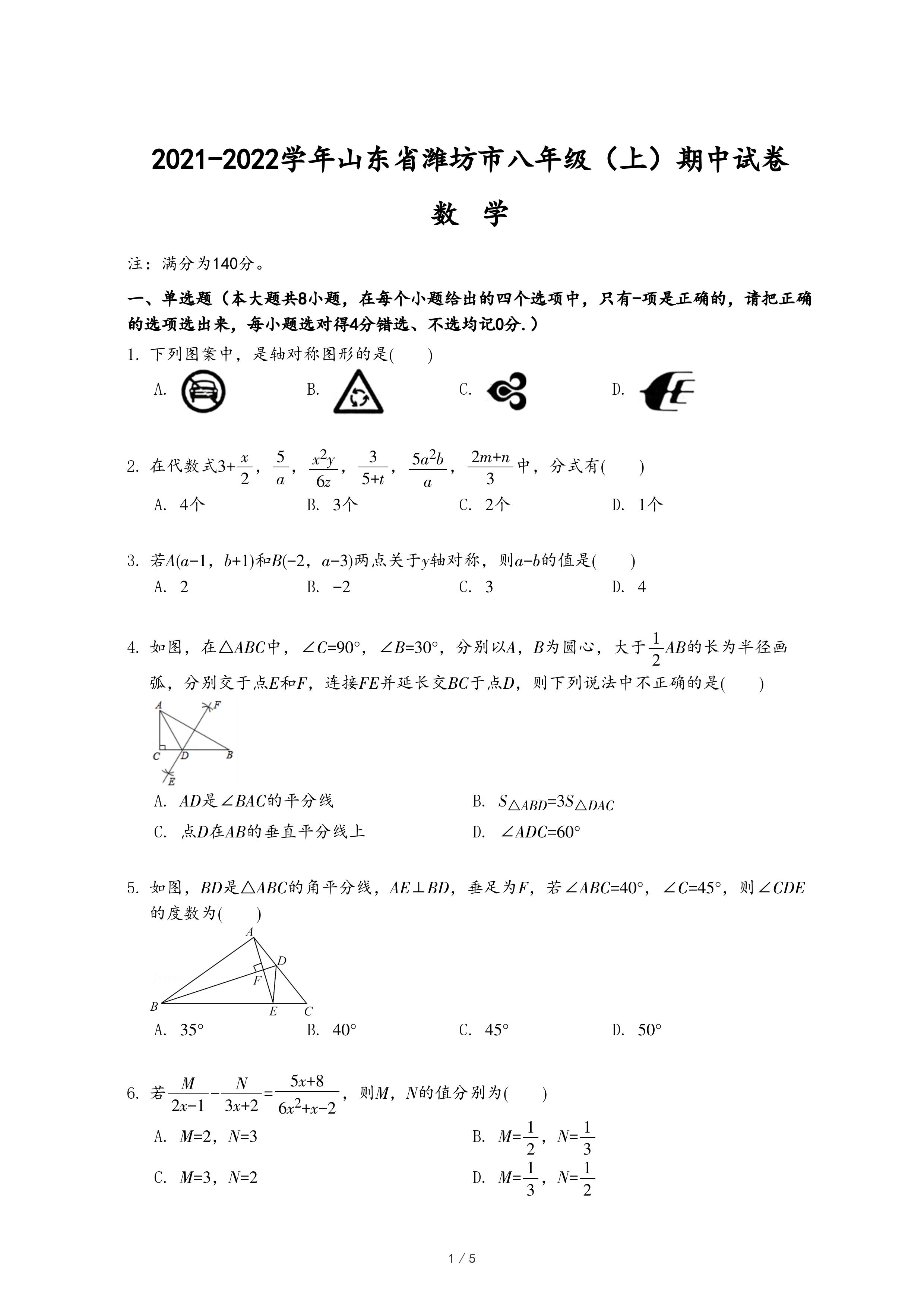1．下列图案中，是轴对称图形的是(　　)
• A.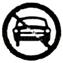• B.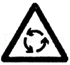• C.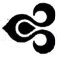• D.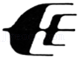2．在代数式3+
 x 2
 5 a
 x2y 6z
 3 5+t
 5a2b a
 2m+n 3

• A. 4个
• B. 3个
• C. 2个
• D. 1个
3．若A(a-1，b+1)和B(-2，a-3)两点关于y轴对称，则a-b的值是(　　)
• A. 2
• B. -2
• C. 3
• D. 4
4．如图，在△ABC中，∠C=90°，∠B=30°，分别以A，B为圆心，大于
 1 2
AB的长为半径画弧，分别交于点E和F，连接FE并延长交BC于点D，则下列说法中不正确的是(　　)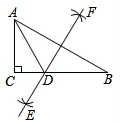• B. SABD=3SDAC
• C. 点D在AB的垂直平分线上
5．如图，BD是△ABC的角平分线，AE⊥BD，垂足为F，若∠ABC=40°，∠C=45°，则∠CDE的度数为(　　)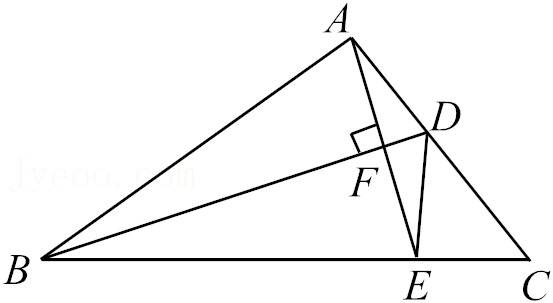• A. 35°
• B. 40°
• C. 45°
• D. 50°
6．
 M 2x-1
-
 N 3x+2
=
 5x+8 6x2+x-2
，则M，N的值分别为(　　)
• A. M=2，N=3
• B. M=
 1 2
，N=
 1 3
• C. M=3，N=2
• D. M=
 1 3
，N=
 1 2

7．如图，把△ABC沿线段DE折叠，使点B落在点F处，若AC∥DE，∠A=70°，AB=AC，则∠CEF的度数为(　　)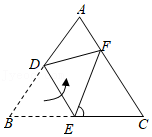• A. 40°
• B. 60°
• C. 70°
• D. 80°
8．已知y=
 x 1-2x
，则
 2x-3xy-2y y-xy-x
=(　　)
• A. -
 1 3
• B. -7
• C. -
 7 3
• D. -5
9．如图，若要判断△ACD≌△ABE，则需要添加的条件是(　　)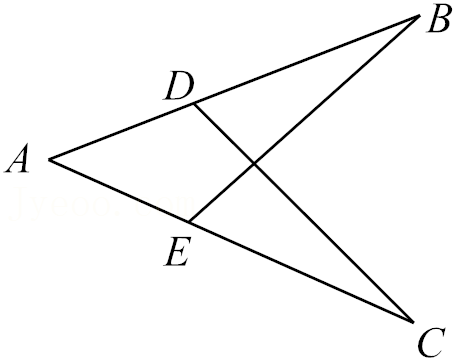• D. AC=AB，CD=BE
10．对于分式
 3-|m| m+3
，下列说法正确的是(　　)
• A. 当m=0时分式无意义
• B. 当m=3时分式的值为0
• C. 当m=-3时分式的值为0
• D. 当m=-2时分式的值为1
11．下列变形正确的是(　　)
• A.
 a b
=
 ab b2

• B.
 a -b-c
=
 -a b-c

• C.
 -a2+2a-1 a2-1
=
 -a+1 a+1

• D.
 x y
=
 x+2 y+2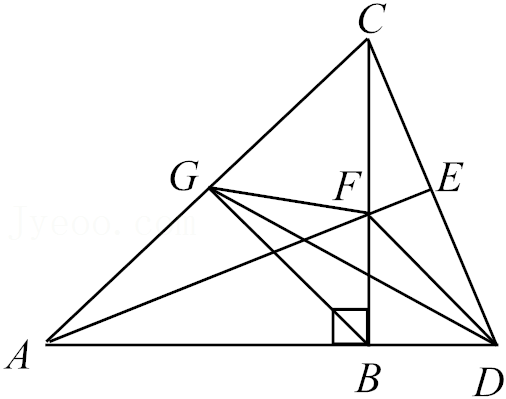• A. AF=2CF
• B. ∠BCD=∠CAE
• C. 点G为AC的中点
• D. AB=BD+DE
13．已知三张卡片上面分别写有6，x-1，x2-1，从中任选两张卡片，组成一个最简分式为       ．(写出一个分式即可)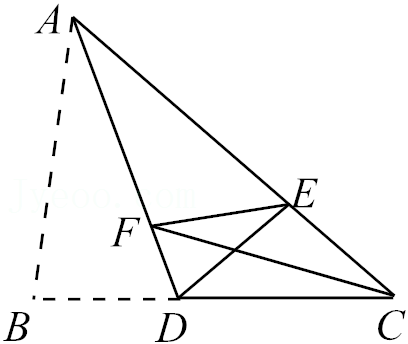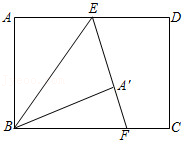16．已知|ab-2|+|a-1|=0，则
 1 ab
+
 1 (a+1)(b+1)
+
 1 (a+2)(b+2)
+⋯+
 1 (a+2021)(b+2021)
=
17．计算：
(1)
 12 m2-9
+
 2 3-m

(2)
 a2-2a+1 a2-a
÷(a-
 1 a
)；
(3)
 x2y x2-y2
÷(
 1 x+y
+
 1 x-y
)．
18．先化简(
 12x x-3
+x-3)÷(
 x2+6x+9 3x2-9x
)，然后从0≤x≤3的范围内选择一个合适的整数作为x的值代入求值．
19．已知：如图，点E，F在BD上，且AE=CF，BF=DE，∠AEB=∠CFD．求证：AC与BD互相平分．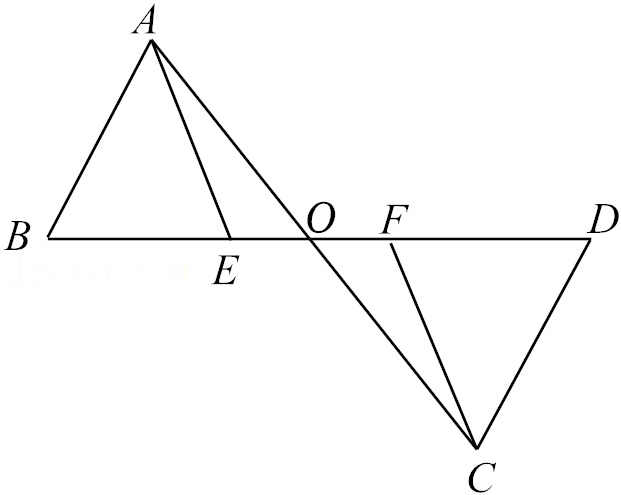20．如图，△ABC中，∠ACB=90°，AE平分∠BAC，DE⊥AB于D．
(1)若∠BAC=48°，求∠DEA的度数；
(2)求证：直线AE是线段CD的垂直平分线．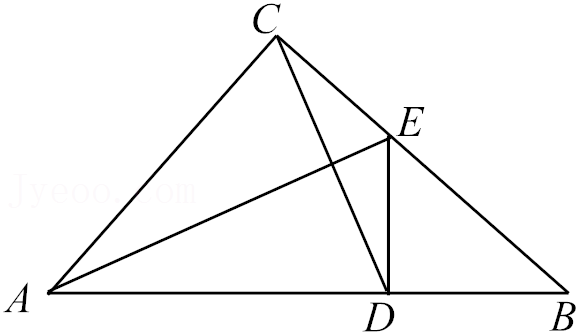21．如图，在所给正方形网格图中完成下列各题：
(1)作△ABC关于y轴的对称图形△A1B1C1
(2)请直接写出点A1，B1，C1的坐标；
(3)在y轴上找一点P使得PA+PB最小，画出点P所在的位置．(保留作图痕迹)